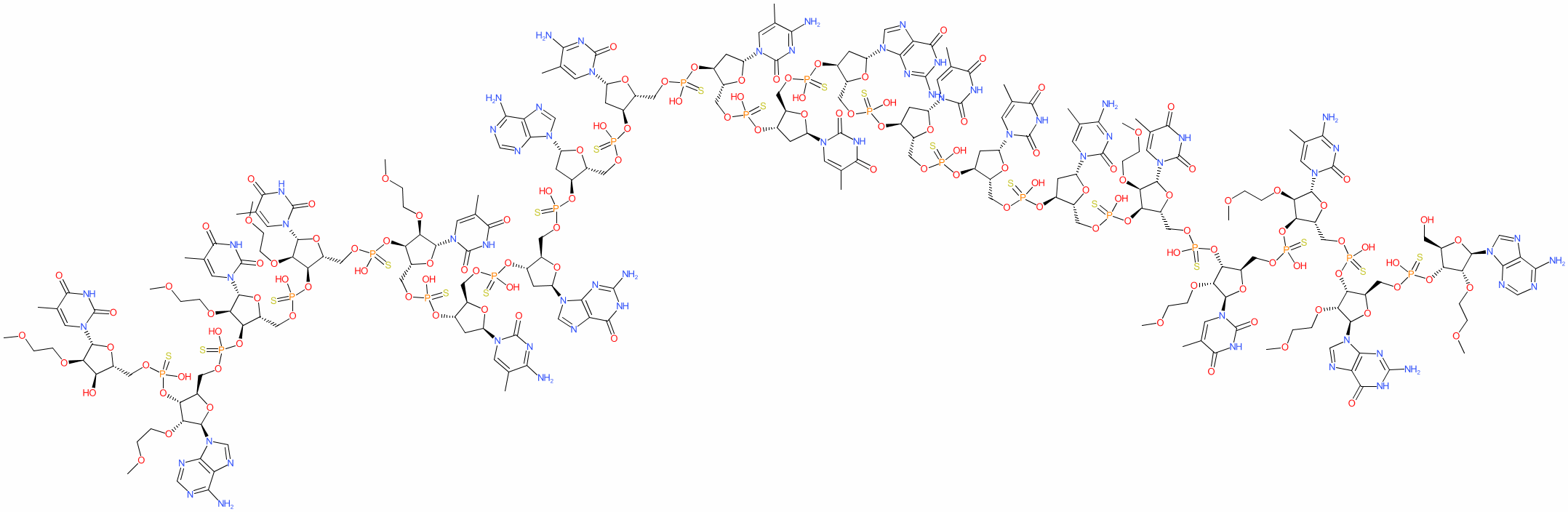#### GtoPdb Ligand ID: 8681

Synonyms: APOC-III antisense inhibitor | ISIS 304801 | ISIS-APOCIIIRx | Waylivra®
volanesorsen is an approved drug (EMA (2019))
Compound class: Synthetic organic
Comment: Volanesorsen is an antisense oligonucleotide (ASO) designed to reduce levels of apolipoprotein C-III (APOC3) in patients with elevated blood triglyceride levels . Reducing triglycerides via APOC3 could be beneficial in patients with inherited hypertriglyceridemia (e.g. those with familial chylomicronemia syndrome, FCS) . The role of APOC3 in hypertriglyceridemia and the effects APOC3 ASO use are reviewed in .
HELM annotation for this RNA (from PubChem) is RNA1{[MOE](A).[sp][MOE](G).[sp][MOE]([5meC]).[sp][MOE](T).[sp][MOE](T).[sp][dR]([5meC]).[sp][dR](T).[sp][dR](T).[sp][dR](G).[sp][dR](T).[sp][dR]([5meC]).[sp][dR]([5meC]).[sp][dR](A).[sp][dR](G).[sp][dR]([5meC]).[sp][MOE](T).[sp][MOE](T).[sp][MOE](T).[sp][MOE](A).[sp][MOE](T)}\$\$\$\$.
2D StructurePhysico-chemical PropertiesHydrogen bond acceptors 130 Hydrogen bond donors 44 Rotatable bonds 156 Topological polar surface area 3216.79 Molecular weight 7160.03 XLogP 6.58 No. Lipinski's rules broken 4
SMILES / InChI / InChIKeyCanonical SMILES COCCO[C@@H]1[C@H](OP(=S)(OC[C@H]2O[C@H](C[C@@H]2OP(=S)(OC[C@H]2O[C@H](C[C@@H]2OP(=S)(OC[C@H]2O[C@H](C[C@@H]2OP(=S)(OC[C@H]2O[C@H](C[C@@H]2OP(=S)(OC[C@H]2O[C@H](C[C@@H]2OP(=S)(OC[C@H]2O[C@H](C[C@@H]2OP(=S)(OC[C@H]2O[C@H](C[C@@H]2OP(=S)(OC[C@H]2O[C@H](C[C@@H]2OP(=S)(OC[C@H]2O[C@H](C[C@@H]2OP(=S)(OC[C@H]2O[C@H](C[C@@H]2OP(=S)(OC[C@H]2O[C@H]([C@@H]([C@@H]2OP(=S)(OC[C@H]2O[C@H]([C@@H]([C@@H]2OP(=S)(OC[C@H]2O[C@H]([C@@H]([C@@H]2OP(=S)(OC[C@H]2O[C@H]([C@@H]([C@@H]2OP(=S)(OC[C@H]2O[C@H]([C@@H]([C@@H]2O)OCCOC)n2cc(C)c(=O)[nH]c2=O)O)OCCOC)n2cnc3c2ncnc3N)O)OCCOC)n2cc(C)c(=O)[nH]c2=O)O)OCCOC)n2cc(C)c(=O)[nH]c2=O)O)OCCOC)n2cc(C)c(=O)[nH]c2=O)O)n2cc(C)c(nc2=O)N)O)n2cnc3c2nc(N)[nH]c3=O)O)n2cnc3c2ncnc3N)O)n2cc(C)c(nc2=O)N)O)n2cc(C)c(nc2=O)N)O)n2cc(C)c(=O)[nH]c2=O)O)n2cnc3c2nc(N)[nH]c3=O)O)n2cc(C)c(=O)[nH]c2=O)O)n2cc(C)c(=O)[nH]c2=O)O)n2cc(C)c(nc2=O)N)O)[C@H](O[C@H]1n1cc(C)c(=O)[nH]c1=O)COP(=S)(O[C@@H]1[C@@H](COP(=S)(O[C@@H]2[C@@H](COP(=S)(O[C@@H]3[C@@H](COP(=S)(O[C@@H]4[C@@H](CO)O[C@H]([C@@H]4OCCOC)n4cnc5c4ncnc5N)O)O[C@H]([C@@H]3OCCOC)n3cnc4c3nc(N)[nH]c4=O)O)O[C@H]([C@@H]2OCCOC)n2cc(C)c(nc2=O)N)O)O[C@H]([C@@H]1OCCOC)n1cc(C)c(=O)[nH]c1=O)O Isomeric SMILES COCCO[C@@H]1[C@H](OP(=S)(OC[C@H]2O[C@H](C[C@@H]2OP(=S)(OC[C@H]2O[C@H](C[C@@H]2OP(=S)(OC[C@H]2O[C@H](C[C@@H]2OP(=S)(OC[C@H]2O[C@H](C[C@@H]2OP(=S)(OC[C@H]2O[C@H](C[C@@H]2OP(=S)(OC[C@H]2O[C@H](C[C@@H]2OP(=S)(OC[C@H]2O[C@H](C[C@@H]2OP(=S)(OC[C@H]2O[C@H](C[C@@H]2OP(=S)(OC[C@H]2O[C@H](C[C@@H]2OP(=S)(OC[C@H]2O[C@H](C[C@@H]2OP(=S)(OC[C@H]2O[C@H]([C@@H]([C@@H]2OP(=S)(OC[C@H]2O[C@H]([C@@H]([C@@H]2OP(=S)(OC[C@H]2O[C@H]([C@@H]([C@@H]2OP(=S)(OC[C@H]2O[C@H]([C@@H]([C@@H]2OP(=S)(OC[C@H]2O[C@H]([C@@H]([C@@H]2O)OCCOC)n2cc(C)c(=O)[nH]c2=O)O)OCCOC)n2cnc3c2ncnc3N)O)OCCOC)n2cc(C)c(=O)[nH]c2=O)O)OCCOC)n2cc(C)c(=O)[nH]c2=O)O)OCCOC)n2cc(C)c(=O)[nH]c2=O)O)n2cc(C)c(nc2=O)N)O)n2cnc3c2nc(N)[nH]c3=O)O)n2cnc3c2ncnc3N)O)n2cc(C)c(nc2=O)N)O)n2cc(C)c(nc2=O)N)O)n2cc(C)c(=O)[nH]c2=O)O)n2cnc3c2nc(N)[nH]c3=O)O)n2cc(C)c(=O)[nH]c2=O)O)n2cc(C)c(=O)[nH]c2=O)O)n2cc(C)c(nc2=O)N)O)[C@H](O[C@H]1n1cc(C)c(=O)[nH]c1=O)COP(=S)(O[C@@H]1[C@@H](COP(=S)(O[C@@H]2[C@@H](COP(=S)(O[C@@H]3[C@@H](COP(=S)(O[C@@H]4[C@@H](CO)O[C@H]([C@@H]4OCCOC)n4cnc5c4ncnc5N)O)O[C@H]([C@@H]3OCCOC)n3cnc4c3nc(N)[nH]c4=O)O)O[C@H]([C@@H]2OCCOC)n2cc(C)c(nc2=O)N)O)O[C@H]([C@@H]1OCCOC)n1cc(C)c(=O)[nH]c1=O)O InChI Key IJUQCWMZCMFFJP-GQSLRNSLSA-N
 References 1. Gaudet D, Brisson D, Tremblay K, Alexander VJ, Singleton W, Hughes SG, Geary RS, Baker BF, Graham MJ, Crooke RM et al.. (2014) Targeting APOC3 in the familial chylomicronemia syndrome. N Engl J Med, 371 (23): 2200-6. [PMID:25470695] 2. Graham MJ, Lee RG, Bell 3rd TA, Fu W, Mullick AE, Alexander VJ, Singleton W, Viney N, Geary R, Su J et al.. (2013) Antisense oligonucleotide inhibition of apolipoprotein C-III reduces plasma triglycerides in rodents, nonhuman primates, and humans. Circ Res, 112 (11): 1479-90. [PMID:23542898] 3. Lee RG, Crosby J, Baker BF, Graham MJ, Crooke RM. (2013) Antisense technology: an emerging platform for cardiovascular disease therapeutics. J Cardiovasc Transl Res, 6 (6): 969-80. [PMID:23856914] 4. Paik J, Duggan S. (2019) Volanesorsen: First Global Approval. Drugs, 79 (12): 1349-1354. [PMID:31301033]